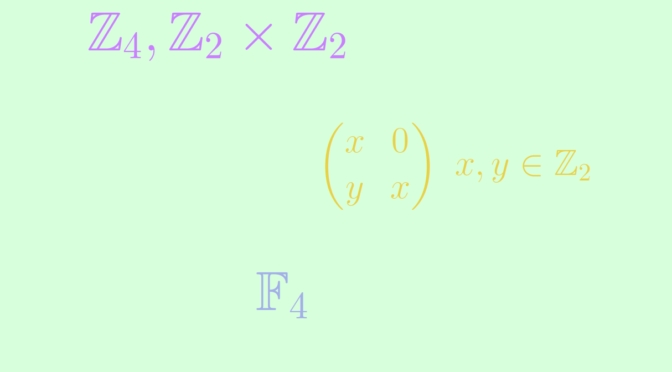Four elements rings

A group with four elements is isomorphic to either the cyclic group $$\mathbb Z_4$$ or to the Klein four-group $$\mathbb Z_2 \times \mathbb Z_2$$. Those groups are commutative. Endowed with the usual additive and multiplicative operations, $$\mathbb Z_4$$ and $$\mathbb Z_2 \times \mathbb Z_2$$ are commutative rings.

Are all four elements rings also isomorphic to either $$\mathbb Z_4$$ or $$\mathbb Z_2 \times \mathbb Z_2$$? The answer is negative. Let’s provide two additional examples of commutative rings with four elements not isomorphic to $$\mathbb Z_4$$ or $$\mathbb Z_2 \times \mathbb Z_2$$.

The first one is the field $$\mathbb F_4$$. $$\mathbb F_4$$ is a commutative ring with four elements. It is not isomorphic to $$\mathbb Z_4$$ or $$\mathbb Z_2 \times \mathbb Z_2$$ as both of those rings have zero divisor. Indeed we have $$2 \cdot 2 = 0$$ in $$\mathbb Z_4$$ and $$(1,0) \cdot (0,1)=(0,0)$$ in $$\mathbb Z_2 \times \mathbb Z_2$$.

A second one is the ring $$R$$ of the matrices $$\begin{pmatrix} x & 0\\ y & x\end{pmatrix}$$ where $$x,y \in \mathbb Z_2$$. One can easily verify that $$R$$ is a commutative subring of the ring $$M_2(\mathbb Z_2)$$. It is not isomorphic to $$\mathbb Z_4$$ as its characteristic is $$2$$. This is not isomorphic to $$\mathbb Z_2 \times \mathbb Z_2$$ either as $$\begin{pmatrix} 0 & 0\\ 1 & 0\end{pmatrix}$$ is a non-zero matrix solution of the equation $$X^2=0$$. $$(0,0)$$ is the only solution of that equation in $$\mathbb Z_2 \times \mathbb Z_2$$.

One can prove that the four rings mentioned above are the only commutative rings with four elements up to isomorphism.